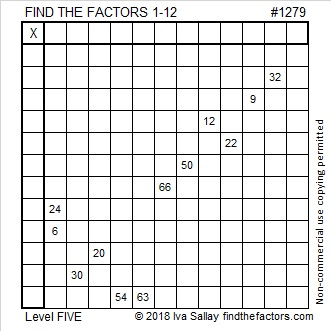# 1279 and Level 5

Can you find the factors for the one and only solution to this puzzle that will make the clues be the products of those factors? Sure you can! Give it a try, and don’t give up no matter what!Print the puzzles or type the solution in this excel file: 12 factors 1271-1280

Now I’ll write a little about the number 1279:

• 1279 is a prime number.
• Prime factorization: 1279 is prime.
• The exponent of prime number 1279 is 1. Adding 1 to that exponent we get (1 + 1) = 2. Therefore 1279 has exactly 2 factors.
• Factors of 1279: 1, 1279
• Factor pairs: 1279 = 1 × 1279
• 1279 has no square factors that allow its square root to be simplified. √1279 ≈ 35.76311

How do we know that 1279 is a prime number? If 1279 were not a prime number, then it would be divisible by at least one prime number less than or equal to √1279 ≈ 35.7. Since 1279 cannot be divided evenly by 2, 3, 5, 7, 11, 13, 17, 19, 23, 29 or 31, we know that 1279 is a prime number.1279 is a prime number that helps us find another VERY big prime number. As OEIS.org informs us, 2¹²⁷⁹ – 1 is also a prime number and is known as a Mersenne Prime.

This site uses Akismet to reduce spam. Learn how your comment data is processed.